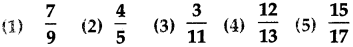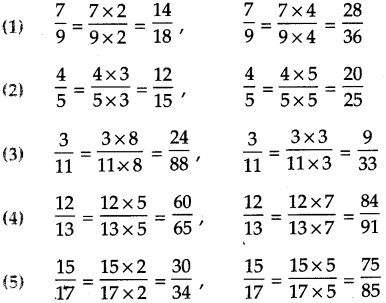# Maharashtra Board Class 5 Maths Solutions Chapter 5 Fractions Problem Set 17

Balbharti Maharashtra Board Class 5 Maths Solutions Chapter 5 Fractions Problem Set 17 Textbook Exercise Important Questions and Answers.

## Maharashtra State Board Class 5 Maths Solutions Chapter 5 Fractions Problem Set 17

Question 1.
Write the proper number in the box.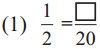Here 20 = 2 x 10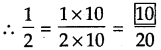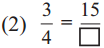Here 15 = 3 x 5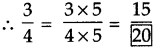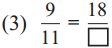Here 18 = 9 x 2
hehce,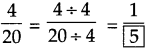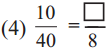Here 40 + 5 = 8,
hence,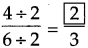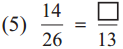Here 26 ÷ 2 = 13,
hence,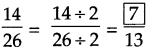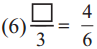Here 6 ÷ 2 = 3,
hence,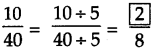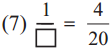Here 4 ÷ 4 = 1,
hence,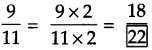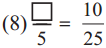Here 25 ÷ 5 = 5,
hence,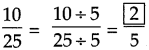Question 2.
Find an equivalent fraction with denominator 18, for each of the following fractions.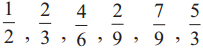Solution: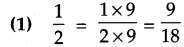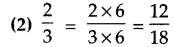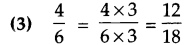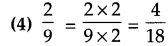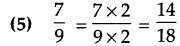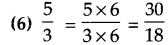Question 3.
Find an equivalent fraction with denominator 5, for each of the following fractions.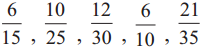Solution: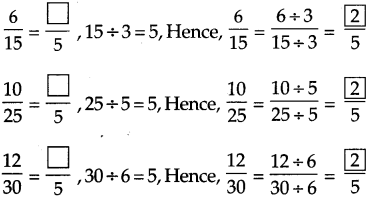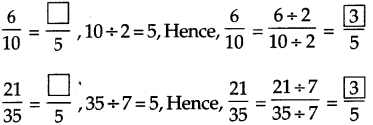Question 4.
From the fractions given below, pair off the equivalent fractions.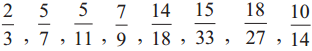Solution: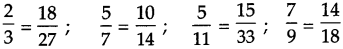Question 5.
Find two equivalent fractions for each of the following fractions.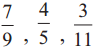Solution: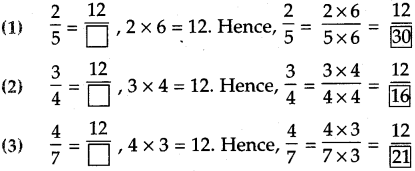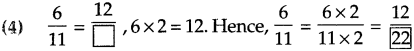Like fractions and unlike fractions

Fractions such as $$\frac{1}{7}, \frac{4}{7}, \frac{6}{7}$$ whose denominators are equal, are called ‘like fractions’.
Fractions such as $$\frac{1}{3}, \frac{4}{8}, \frac{9}{11}$$ which have different denominators are called unlike fractions’.

Converting unlike fractions into like fractions

Example (1) Convert 5/6 and 7/9 into like fractions.
Here, we must find a common multiple for the numbers 6 and 9.
Multiples of 6 : 6, 12, 18, 24, 30, 36, ……..
Multiples of 9 : 9, 18, 27, 36, 45 ……..
Here, the number 18 is a multiple of both 6 and 9. So, let us make 18 the denominator of both fractions.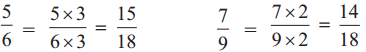Thus, 15/18 and 1418 are like fractions, respectively equivalent to 5/6 and 7/9.
Here, 18 is a multiple of both 6 and 9. We could also choose numbers like 36 and 54 as the common denominators.

Example (2) Convert 4/8 and 5/16 into like fractions.
As 16 is twice 8, it is easy to make 16 the common denominator.
$$\frac{4}{8}=\frac{4 \times 2}{8 \times 2}=\frac{8}{16}$$ Thus, 8/16 and 5/16 are the required like fractions.

Example (3) Find a common denominator for 4/7 and 3/4.
The number 28 is a multiple of both 7 and 4. So, make 28 the common denominator.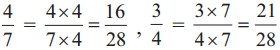. Therefore, 16/28 and 21/28 are the required like fractions.